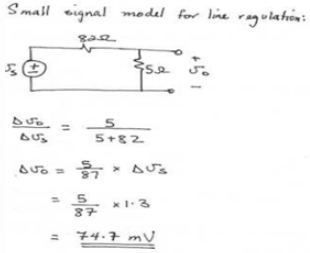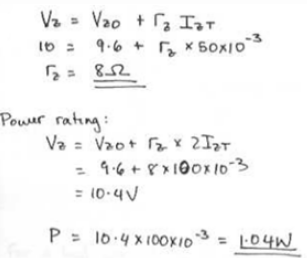Courses

# Test: Zener Diode

## 10 Questions MCQ Test Analog Electronics | Test: Zener Diode

Description
This mock test of Test: Zener Diode for Electrical Engineering (EE) helps you for every Electrical Engineering (EE) entrance exam. This contains 10 Multiple Choice Questions for Electrical Engineering (EE) Test: Zener Diode (mcq) to study with solutions a complete question bank. The solved questions answers in this Test: Zener Diode quiz give you a good mix of easy questions and tough questions. Electrical Engineering (EE) students definitely take this Test: Zener Diode exercise for a better result in the exam. You can find other Test: Zener Diode extra questions, long questions & short questions for Electrical Engineering (EE) on EduRev as well by searching above.
QUESTION: 1

### Zener diodes are also known as

Solution:

Zener diodes are used as voltage regulators but they aren’t called voltage regulators. They are called breakdown diodes since they operate in breakdown region.

QUESTION: 2

### Which of the following is true about the resistance of a Zener diode?

Solution:

All of the statements are true for the resistance of the zener diode.

QUESTION: 3

### Which of the following is true about the temperature coefficient or TC of the Zener diode?

Solution:

All of the mentioned are true for the TC of a zener diode.

QUESTION: 4

Which of the following can be used in series with a Zener diode so that combination has almost zero temperature coefficient?

Solution:

If a Zener diode of TC of about -2mV is connected with a forward diode (which has a TC of about +2mV) in series, the combination can be used to obtain a very low (close to zero) TC.

QUESTION: 5

In Zener diode, for currents greater than the knee current, the v-i curve is almost

Solution:

Note that the curve is v-I curve and not an i-v curve.

QUESTION: 6

Zener diodes can be effectively used in voltage regulator. However, they are these days being replaced by more efficient

Solution:

ICs have been widely adapted by the industries over conventional zener diodes as their better replacements for a voltage regulators.

QUESTION: 7

A 9.1-V zener diode exhibits its nominal voltage at a test current of 28 mA. At this current the incremental resistance is specified as 5 Ω. Find VZ0 of the Zener model.

Solution:

VZ = VZo + MZ IZT
9.1 = VZo + 5 * 28 * 10-3
VZo = 8.96v
VZ = VZo + 5IZ = 8.96 * 5IZ.

QUESTION: 8

A shunt regulator utilizing a zener diode with an incremental resistance of 5 Ω is fed through an 82-Ω resistor. If the raw supply changes by 1.0 V, what is the corresponding change in the regulated output voltage?

Solution:QUESTION: 9

A designer requires a shunt regulator of approximately 20 V. Two kinds of Zener diodes are available: 6.8-V devices with rz of 10 Ω and 5.1-V devices with rz of 30 Ω. For the two major choices possible, find the load regulation. In this calculation neglect the effect of the regulator resistance R.

Solution:

Three 6.8v zeners provide 3*6.8 = 20.4v with 3 * 10 =30Ω Resistance, neglecting R, we have
For 5.1 Zeners we need 4 diodes to provide 20.4v with 4 * 30 =120Ω Resistance.